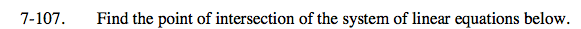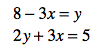### Home > CAAC > Chapter 7 > Lesson 7.3.3 > Problem7-107

7-107.Rearrange the top equation so that it matches the form of the bottom equation.
y − 3x = −8
2y + 3x = 5

Solve the system of equations by eliminating the x's.
y = −3

Now substitute the value for Y back into one of the original equations to solve for X.

$\left(\frac{11}{3},-3\right)$